Angle sum property of a triangle

Chapter 6 Class 7 Triangle and its Properties
Concept wise

Sum of angles of a triangle is 180°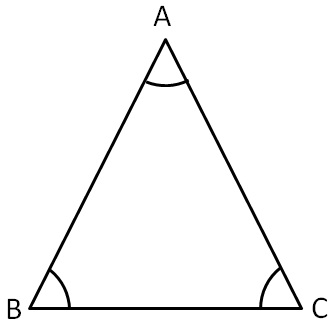Here,

∠A + ∠B + ∠C = 180°

For proof, please check Theorem 6.7

Let’s solve some examples

### Show that sum of angles of the triangle is 180°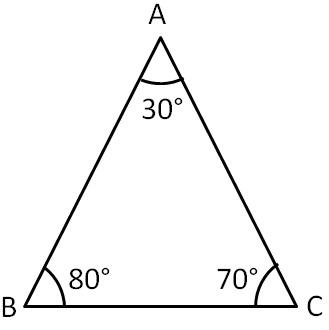Sum of all angles of triangle = ∠A + ∠B + ∠C

= 30° + 80° + 70°

= 110° + 70°

= 180°

### Show that sum of angles of the triangle is 180°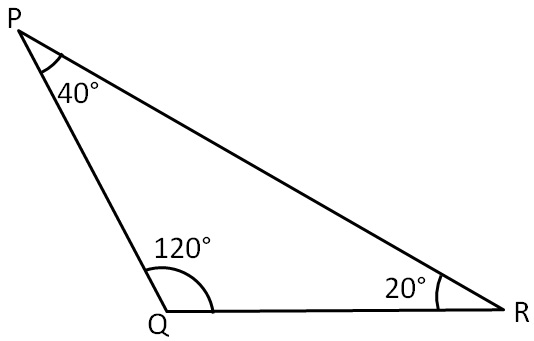Sum of all angles of triangle = ∠P + ∠Q + ∠R

= 40° + 120° + 20°

= 180°

### Show that sum of angles of the triangle is 180°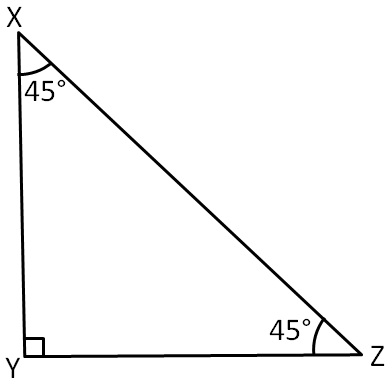Sum of all angles of triangle = ∠X + ∠Y + ∠Z

= 45° + 90° + 45°

= 180°

### Find the missing angle of following triangles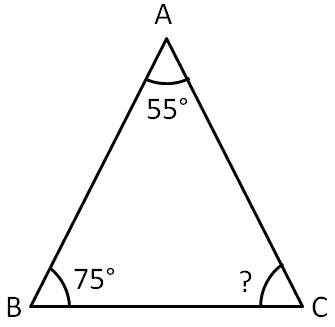In ∆ABC,

∠A + ∠B + ∠C = 180°               (Angle sum property of triangle)

55° + 75° + ∠C = 180°

130° + ∠C = 180°

∠C = 180°  − 130°

∠C = 50°

### Find the missing angle of following triangles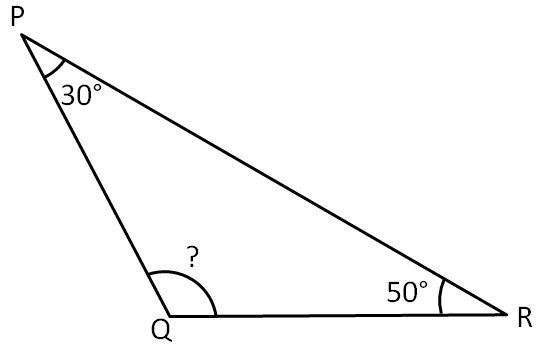In ∆PQR,

∠P + ∠Q + ∠R = 180°       (Angle sum property of triangle)

30° + ∠Q + 50° = 180°

∠Q + 80° = 180°

∠Q = 180°  − 80°

∠Q = 100°

### Find the missing angle of following triangles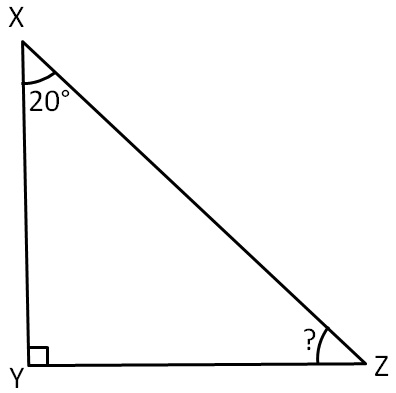In ∆XYZ,

∠X + ∠Y + ∠Z = 180°         (Angle sum property of triangle)

20° + 90° + ∠Z  = 180°

110° + ∠Z = 180°

∠Z = 180° − 110°

∠Z = 70°

Get live Maths 1-on-1 Classs - Class 6 to 12